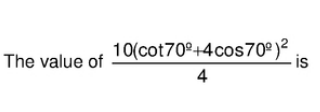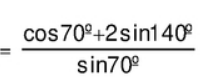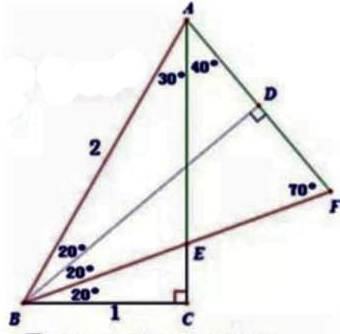# Doubt from trigonometryWhat to do after this?How sin80 have came in third step, which formulae is applied by you?

Identity is
sinA+sinB=2sin\frac{A+B}2cos\frac{A-B}2
and then
cos(90-\theta)=sin\theta

Proof Without Words::

Given \cot(70^\circ)+4\cos(70^\circ)=\tan(20^\circ)+4\sin(20^\circ)From Diagram

\Longrightarrow \text{AC=AE+CE=AF+CE.}